# 24.1.4. Interpolation¶

## 24.1.4.1. Heatmap (kernel density estimation)¶

Creates a density (heatmap) raster of an input point vector layer using kernel density estimation.

The density is calculated based on the number of points in a location, with larger numbers of clustered points resulting in larger values. Heatmaps allow easy identification of hotspots and clustering of points.

### 24.1.4.1.1. Parameters¶

Label

Name

Type

Description

Point layer

`INPUT`

[vector: point]

Point vector layer to use for the heatmap

`RADIUS`

[number]

Default: 100.0

Heatmap search radius (or kernel bandwidth) in map units. The radius specifies the distance around a point at which the influence of the point will be felt. Larger values result in greater smoothing, but smaller values may show finer details and variation in point density.

Output raster size

`PIXEL_SIZE`

[number]

Default: 0.1

Pixel size of the output raster layer in layer units.

In the GUI, the size can be specified by the number of rows (`Number of rows`) / columns (`Number of columns`) or the pixel size( `Pixel Size X` / `Pixel Size Y`). Increasing the number of rows or columns will decrease the cell size and increase the file size of the output raster. The values in `Rows`, `Columns`, `Pixel Size X` and `Pixel Size Y` will be updated simultaneously - doubling the number of rows will double the number of columns, and the cell size will be halved. The extent of the output raster will remain the same (approximately).

Optional

`RADIUS_FIELD`

[tablefield: numeric]

Sets the search radius for each feature from an attribute field in the input layer.

Weight from field

Optional

`WEIGHT_FIELD`

[tablefield: numeric]

Allows input features to be weighted by an attribute field. This can be used to increase the influence certain features have on the resultant heatmap.

Kernel shape

`KERNEL`

[enumeration]

Default: 0

Controls the rate at which the influence of a point decreases as the distance from the point increases. Different kernels decay at different rates, so a triweight kernel gives features greater weight for distances closer to the point then the Epanechnikov kernel does. Consequently, triweight results in “sharper” hotspots and Epanechnikov results in “smoother” hotspots.

• 0 — Quartic

• 1 — Triangular

• 2 — Uniform

• 3 — Triweight

• 4 — Epanechnikov

Decay ratio (Triangular kernels only)

Optional

`DECAY`

[number]

Default: 0.0

Can be used with Triangular kernels to further control how heat from a feature decreases with distance from the feature.

• A value of 0 (=minimum) indicates that the heat will be concentrated in the center of the given radius and completely extinguished at the edge.

• A value of 0.5 indicates that pixels at the edge of the radius will be given half the heat as pixels at the center of the search radius.

• A value of 1 means the heat is spread evenly over the whole search radius circle. (This is equivalent to the ‘Uniform’ kernel.)

• A value greater than 1 indicates that the heat is higher towards the edge of the search radius than at the center.

Output value scaling

`OUTPUT_VALUE`

[enumeration]

Default: Raw

Allow to change the values of the output heatmap raster. One of:

• 0 — Raw

• 1 — Scaled

Heatmap

`OUTPUT`

[raster]

Default: `[Save to temporary file]`

Specify the output raster layer with kernel density values. One of:

• Save to a Temporary File

• Save to File…

The file encoding can also be changed here.

### 24.1.4.1.2. Outputs¶

Label

Name

Type

Description

Heatmap

`OUTPUT`

[raster]

Raster layer with kernel density values

### 24.1.4.1.3. Example: Creating a Heatmap¶

For the following example, we will use the `airports` vector point layer from the QGIS sample dataset (see Downloading sample data). Another excellent QGIS tutorial on making heatmaps can be found at http://qgistutorials.com.

In Fig. 24.2, the airports of Alaska are shown.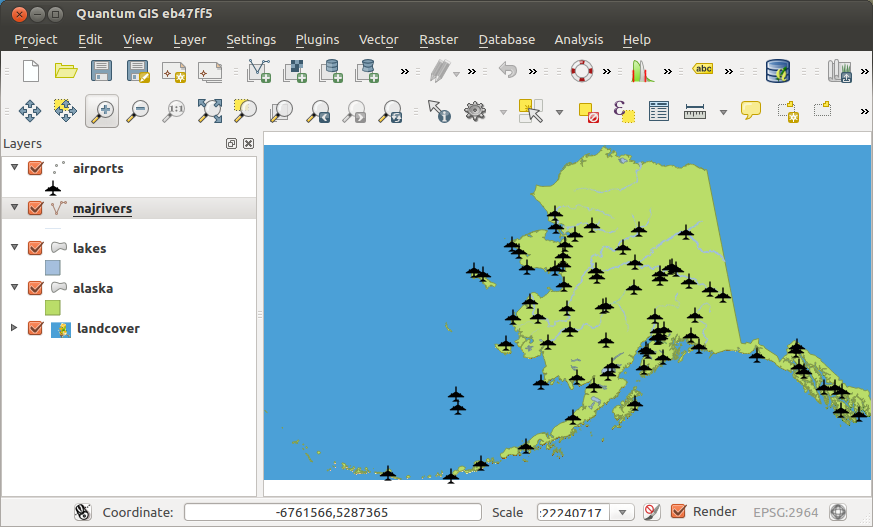1. Open the Heatmap (Kernel Density Estimation) algorithm from the QGIS Interpolation group

2. In the Point layer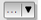field, select `airports` from the list of point layers loaded in the current project.

3. Change the Radius to `1000000` meters.

4. Change the Pixel size X to `1000`. The Pixel size Y, Rows and Columns will be automatically updated.

5. Click on Run to create and load the airports heatmap (see Fig. 24.4).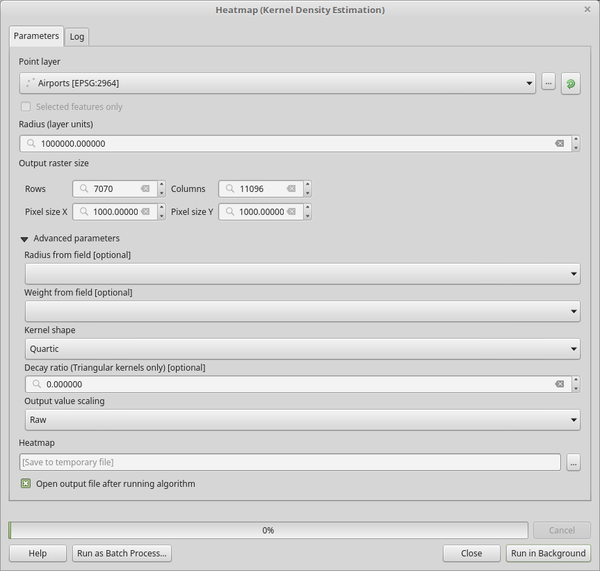Fig. 24.3 The Heatmap Dialog

QGIS will generate the heatmap and add it to your map window. By default, the heatmap is shaded in greyscale, with lighter areas showing higher concentrations of airports. The heatmap can now be styled in QGIS to improve its appearance.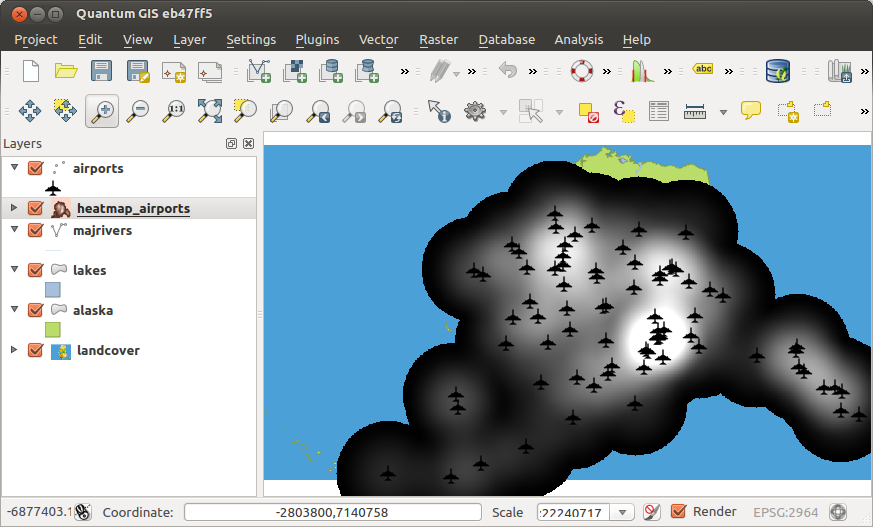1. Open the properties dialog of the `heatmap_airports` layer (select the layer `heatmap_airports`, open the context menu with the right mouse button and select Properties).

2. Select the Symbology tab.

3. Change the Render typeto ‘Singleband pseudocolor’.

4. Select a suitable Color ramp, for instance `YlOrRd`.

5. Click the Classify button.

6. Press OK to update the layer.

The final result is shown in Fig. 24.5.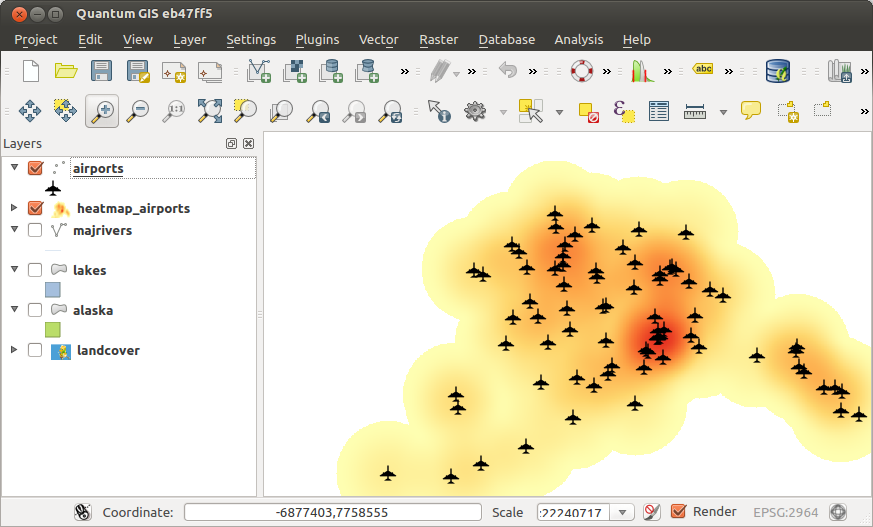Fig. 24.5 Styled heatmap of airports of Alaska

### 24.1.4.1.4. Python code¶

Algorithm ID: `qgis:heatmapkerneldensityestimation`

```import processing
processing.run("algorithm_id", {parameter_dictionary})
```

The algorithm id is displayed when you hover over the algorithm in the Processing Toolbox. The parameter dictionary provides the parameter NAMEs and values. See Using processing algorithms from the console for details on how to run processing algorithms from the Python console.

## 24.1.4.2. IDW Interpolation¶

Generates an Inverse Distance Weighted (IDW) interpolation of a point vector layer.

Sample points are weighted during interpolation such that the influence of one point relative to another declines with distance from the unknown point you want to create.

The IDW interpolation method also has some disadvantages: the quality of the interpolation result can decrease, if the distribution of sample data points is uneven.

Furthermore, maximum and minimum values in the interpolated surface can only occur at sample data points.

### 24.1.4.2.1. Parameters¶

Label

Name

Type

Description

Input layer(s)

`INTERPOLATION_DATA`

[string]

Vector layer(s) and field(s) to use for the interpolation, coded in a string (see the `ParameterInterpolationData` class in InterpolationWidgets for more details).

The following GUI elements are provided to compose the interpolation data string:

• Vector layer [vector: any]

• Interpolation attribute [tablefield: numeric]: Attribute to use in the interpolation

• Use Z-coordinate for interpolation [boolean]: Uses the layer’s stored Z values (Default: False)

For each of the added layer-field combinations, a type can be chosen:

• Points

• Structured lines

• Break lines

In the string, the layer-field elements are separated by `'::|::'`. The sub-elements of the layer-field elements are separated by `'::~::'`.

Distance coefficient P

`DISTANCE_COEFFICIENT`

[number]

Default: 2.0

Sets the distance coefficient for the interpolation. Minimum: 0.0, maximum: 100.0.

Extent (xmin, xmax, ymin, ymax)

`EXTENT`

[extent]

Extent of the output raster layer. You have to declare the output extent by either choosing it from the map canvas, selecting it from another layer or type it manually.

Output raster size

`PIXEL_SIZE`

[number]

Default: 0.1

Pixel size of the output raster layer in layer units.

In the GUI, the size can be specified by the number of rows (`Number of rows`) / columns (`Number of columns`) or the pixel size( `Pixel Size X` / `Pixel Size Y`). Increasing the number of rows or columns will decrease the cell size and increase the file size of the output raster. The values in `Rows`, `Columns`, `Pixel Size X` and `Pixel Size Y` will be updated simultaneously - doubling the number of rows will double the number of columns, and the cell size will be halved. The extent of the output raster will remain the same (approximately).

Interpolated

`OUTPUT`

[raster]

Default: `[Save to temporary file]`

Raster layer of interpolated values. One of:

• Save to a Temporary File

• Save to File…

The file encoding can also be changed here.

### 24.1.4.2.2. Outputs¶

Label

Name

Type

Description

Interpolated

`OUTPUT`

[raster]

Raster layer of interpolated values

### 24.1.4.2.3. Python code¶

Algorithm ID: `qgis:idwinterpolation`

```import processing
processing.run("algorithm_id", {parameter_dictionary})
```

The algorithm id is displayed when you hover over the algorithm in the Processing Toolbox. The parameter dictionary provides the parameter NAMEs and values. See Using processing algorithms from the console for details on how to run processing algorithms from the Python console.

## 24.1.4.3. Line Density¶

Calculates for each raster cell, the density measure of linear features within a circular neighbourhood. This measure is obtained by summing all the line segments intersecting the circular neighbourhood and dividing this sum by the area of such neighbourhood. A weighting factor can be applied to the line segments.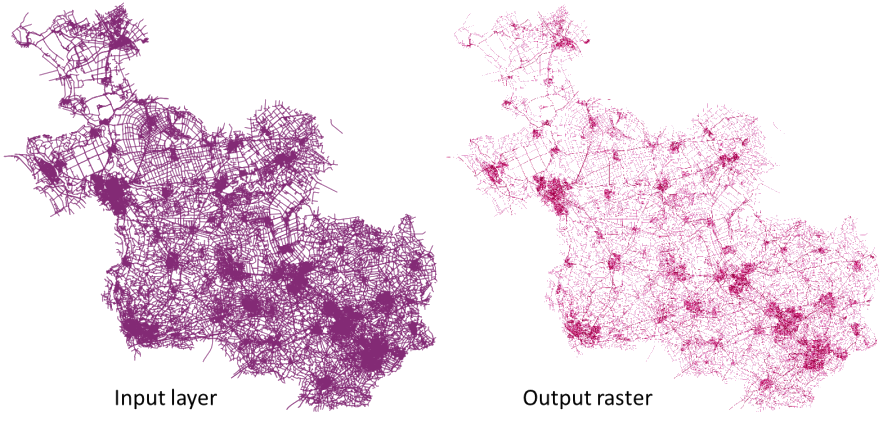Fig. 24.6 Line density example. Input layer source: Roads Overijssel - The Netherlands (OSM).

### 24.1.4.3.1. Parameters¶

Label

Name

Type

Description

Input line layer

`INPUT`

[vector: any]

Input vector layer containing line features

Weight field

`WEIGHT`

[number]

Field of the layer containing the weight factor to use during the calculation

`RADIUS`

[number]

Default: 10

Radius of the circular neighbourhood. Units can be specified here.

Pixel size

`PIXEL_SIZE`

[number]

Default: 10

Pixel size of the output raster layer in layer units. The raster has square pixels.

Line density raster

`OUTPUT`

[raster]

Default: `[Save to temporary file]`

The output as a raster layer. One of:

• Save to a Temporary File

• Save to File…

The file encoding can also be changed here.

### 24.1.4.3.2. Outputs¶

Label

Name

Type

Description

Line density raster

`OUTPUT`

[raster]

The output line density raster layer.

### 24.1.4.3.3. Python code¶

Algorithm ID: `qgis:linedensity`

```import processing
processing.run("algorithm_id", {parameter_dictionary})
```

The algorithm id is displayed when you hover over the algorithm in the Processing Toolbox. The parameter dictionary provides the parameter NAMEs and values. See Using processing algorithms from the console for details on how to run processing algorithms from the Python console.

## 24.1.4.4. TIN Interpolation¶

Generates a Triangulated Irregular Network (TIN) interpolation of a point vector layer.

With the TIN method you can create a surface formed by triangles of nearest neighbor points. To do this, circumcircles around selected sample points are created and their intersections are connected to a network of non overlapping and as compact as possible triangles. The resulting surfaces are not smooth.

The algorithm creates both the raster layer of the interpolated values and the vector line layer with the triangulation boundaries.

### 24.1.4.4.1. Parameters¶

Label

Name

Type

Description

Input layer(s)

`INTERPOLATION_DATA`

[string]

Vector layer(s) and field(s) to use for the interpolation, coded in a string (see the `ParameterInterpolationData` class in InterpolationWidgets for more details).

The following GUI elements are provided to compose the interpolation data string:

• Vector layer [vector: any]

• Interpolation attribute [tablefield: numeric]: Attribute to use in the interpolation

• Use Z-coordinate for interpolation [boolean]: Uses the layer’s stored Z values (Default: False)

For each of the added layer-field combinations, a type can be chosen:

• Points

• Structured lines

• Break lines

In the string, the layer-field elements are separated by `'::|::'`. The sub-elements of the layer-field elements are separated by `'::~::'`.

Interpolation method

`METHOD`

[enumeration]

Default: 0

Set the interpolation method to be used. One of:

• Linear

• Clough-Toucher (cubic)

Extent (xmin, xmax, ymin, ymax)

`EXTENT`

[extent]

Extent of the output raster layer. You have to declare the output extent by either choosing it from the map canvas, selecting it from another layer or type it manually.

Output raster size

`PIXEL_SIZE`

[number]

Default: 0.1

Pixel size of the output raster layer in layer units.

In the GUI, the size can be specified by the number of rows (`Number of rows`) / columns (`Number of columns`) or the pixel size( `Pixel Size X` / `Pixel Size Y`). Increasing the number of rows or columns will decrease the cell size and increase the file size of the output raster. The values in `Rows`, `Columns`, `Pixel Size X` and `Pixel Size Y` will be updated simultaneously - doubling the number of rows will double the number of columns, and the cell size will be halved. The extent of the output raster will remain the same (approximately).

Interpolated

`OUTPUT`

[raster]

Default: `[Save to temporary file]`

The output TIN interpolation as a raster layer. One of:

• Save to a Temporary File

• Save to File…

The file encoding can also be changed here.

Triangulation

`TRIANGULATION`

[vector: line]

Default: `[Skip output]`

The output TIN as a vector layer. One of:

• Skip Output

• Create Temporary Layer (`TEMPORARY_OUTPUT`)

• Save to File…

• Save to Geopackage…

• Save to PostGIS Table…

### 24.1.4.4.2. Outputs¶

Label

Name

Type

Description

Interpolated

`OUTPUT`

[raster]

The output TIN interpolation as a raster layer

Triangulation

`TRIANGULATION`

[vector: line]

The output TIN as a vector layer.

### 24.1.4.4.3. Python code¶

Algorithm ID: `qgis:tininterpolation`

```import processing
processing.run("algorithm_id", {parameter_dictionary})
```

The algorithm id is displayed when you hover over the algorithm in the Processing Toolbox. The parameter dictionary provides the parameter NAMEs and values. See Using processing algorithms from the console for details on how to run processing algorithms from the Python console.